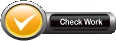Name:    3rd Grade Measurement & DataGeometric measurement: understand concepts of area and relate area to multiplication and to addition.  Standard 5 & 6, Test 1

Multiple Choice
Identify the choice that best completes the statement or answers the question.

1.The yellow square represent one _________ when measuring area.
 a. one length c. one square side b. one square unit d. one unit

2.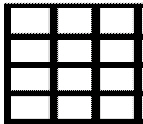Which answer below is correct when measuring the area of the large square?
 a. there are four lengths by three lengths in the larger square c. there are four units in the larger square b. there are twelve square sides in the larger square d. there are twelve square units in the large square

Numeric Response

3.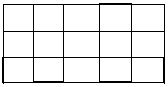How many square units does this rectangle have?

4.How many square units does this rectangle have?

5.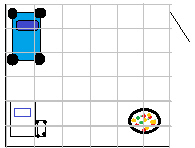Silvia wanted to put carpet in her bedroom.  She had to know how many square feet she needed to buy.  How many square feet of carpet does Silvia need to carpet her room?

6.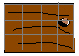Fred measured a board for a project he was doing.  The board was the right size.  How many square inches is Fred’s board?

7.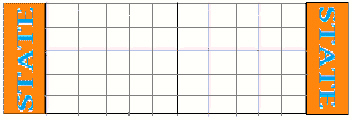The football team is getting new turf.  The turf is cut into squares of 10 yards by 10 yeards.  How many square units of turf does the team need to do the whole field?

8.Albert was designing a box for his baby snake.  His design was diveded into square cm’s.  How many square cm’s is the top of the box Albert wants to put his snake in?